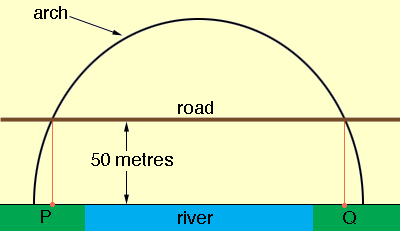SEARCH HOMEMath Central Quandaries & QueriesQuestion from Taranjeet, a student: My teacher has given us bridge with only one measurement. From the river to the roadway is 50 metres in length (vertically) The question he wants us to find out is. What is the distance between the vertical supports. He has said that: At a horizontal distance of 'x' metres from the foot of the arch the height of the arch, the height of the arch above the river 'h' metres is given by: h=-1/40(x squared) = 3x I don't understand how to find the distance between the support beams. Thank youTaranjeet,

I'm not sure I understand either but I'll show you what I think is the situation. First I think there is a typo in what you sent. I think the function should be

h = - 1/40 x2 + 3x

This is a parabola that opens downward and crosses the x-axis at x = 0 metres and x = 120 metres. This gives the height of the arch above the river so the endpoints of the arch are on the x-axis. The road is 50 metres above the river so the image I have isIf this is the correct interpretation then you can find the x-coordinates of the points P and Q. These are the values of x for which

- 1/40 x2 + 3x = 50

I don't know if this is where the vertical supports are placed.

I hope this helps,
PennyMath Central is supported by the University of Regina and The Pacific Institute for the Mathematical Sciences.